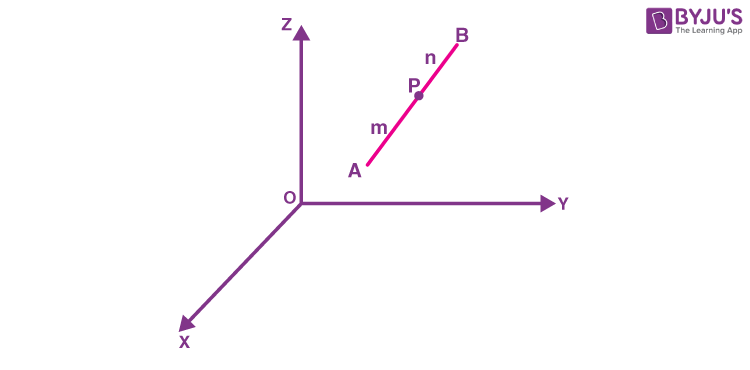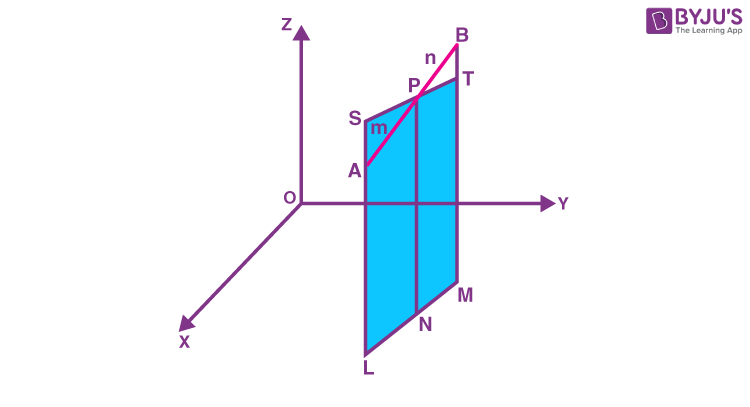# Section Formula in 3D

In two dimension geometry, the concept of section formula is implemented to find the coordinates of a point dividing a line segment internally in a specific ratio. In order to locate the position of a point in space, we require a coordinate system. After choosing a fixed coordinate system in three dimensions, the coordinates of any point P in that system can be given. In case of a rectangular coordinate system, it is given by an ordered 3-tuple (x, y, z). Also, if the coordinates (x, y, z) is already known, then we can easily locate the point P in space. The concept of section formula can be extended to three-dimension geometry as well as to determine the coordinates of a point dividing a line in a certain ratio.

## Section Formula

Let us consider two points A (x1, y1, z1) and B(x2, y2, z2). Consider a point P(x, y, z) dividing AB in the ratio m:n as shown in the figure given below.To determine the coordinates of the point P, the following steps are followed:• Draw AL, PN, and BM perpendicular to XY plane such that AL || PN || BM as shown above.
• The points L, M and N lie on the straight line formed due to the intersection of a plane containing AL, PN and BM and XY- plane.
• From point P, a line segment ST is drawn such that it is parallel to LM.
• ST intersects AL externally at S, and it intersects BM at T internally.

Since ST is parallel to LM and AL || PN || BM, therefore, the quadrilaterals LNPS and NMTP qualify as parallelograms.

Also, ∆ASP ~∆BTP therefore,

$$\begin{array}{l}\large \frac{m}{n} = \frac{AP}{BP} = \frac{AS}{BT} = \frac{SL – AL}{BM – TM} = \frac{NP – AL}{BM – PN} = \frac{z – z_{1}}{z_{2} – z}\end{array}$$

Rearranging the above equation we get,

$$\begin{array}{l}z=\large \frac{mz_{2}+ nz_{1}}{m + n}\end{array}$$

The above procedure can be repeated by drawing perpendiculars to XZ and YZ- planes to get the x and y coordinates of the point P that divides the line segment AB in the ratio m:n internally.

$$\begin{array}{l}\large x = \frac{mx_{2}+ nx_{1}}{m+n}, y = \frac{my_{2}+ ny_{1}}{m+n}\end{array}$$

### Sectional Formula (Internally)

Thus, the coordinates of the point P(x, y, z) dividing the line segment joining the points A(x1, y1, z1) and B(x2, y2, z2) in the ratio m:n internally are given by:

$$\begin{array}{l}\large \left ( \frac{mx_{2}+ nx_{1}}{m+n}, \frac{my_{2}+ ny_{1}}{m+n}, \frac{mz_{2}+ nz_{1}}{m+n} \right )\end{array}$$

### Sectional Formula (Externally)

If the given point P divides the line segment joining the points A(x1, y1, z1) and B(x2, y2, z2) externally in the ratio m:n, then the coordinates of P are given by replacing n with –n as:

$$\begin{array}{l}\large \left ( \frac{mx_{2}- nx_{1}}{m-n}, \frac{my_{2}- ny_{1}}{m-n}, \frac{mz_{2}- nz_{1}}{m-n} \right )\end{array}$$

This represents the section formula for three dimension geometry.

If the point P divides the line segment joining points A and B internally in the ratio k:1, then the coordinates of point P will be

$$\begin{array}{l}\large \left ( \frac{kx_{2}+ x_{1}}{k+1}, \frac{ky_{2}+ y_{1}}{k+1}, \frac{kz_{2}+ z_{1}}{k+1} \right )\end{array}$$

What if the point P dividing the line segment joining points A(x1, y1, z1) and B(x2, y2, z2) is the midpoint of line segment AB?

In that case, if P is the midpoint, then P divides the line segment AB in the ratio 1:1, i.e. m=n=1.

Coordinates of point P will be given as:

$$\begin{array}{l}\large \left ( \frac{1 \times x_{2}+ 1 \times x_{1}}{1+1}, \frac{1 \times y_{2}+ 1 \times y_{1}}{1+1}, \frac{1 \times z_{2}+ 1 \times z_{1}}{1+1} \right )\end{array}$$

Therefore, the coordinates of the midpoint of line segment joining points A(x1, y1, z1) and B(x2, y2, z2) are given by,

$$\begin{array}{l}\large \left ( \frac{x_{2}+ x_{1}}{2}, \frac{y_{2}+ y_{1}}{2}, \frac{z_{2}+ z_{1}}{2} \right )\end{array}$$

## Section Formula in 3D Examples

Go through the below examples to understand the concept of section formula in a three-dimensional plane.

Example 1:

Determine the coordinate points which divide the line segment that joins the point (1, -2, 3) and (3, 4, -5) in the ratio of 2:3 internally and externally.

Solution:

Assume that the point P(x, y, z) divides the line segment that joins A(1, -2, 3) and B(3, 4, -5) internally in the ratio of 2:3.

Here, m=2, and n=3

Now, substitute the values in the section formula,

⇒ [(mx2+nx1)/m+n, (my2+ny1)/m+n, (mz2+nz1)/m+n ]

⇒ [(2(3)+3(1))/2+3,(2(4)+3(-2))/2+3, (2(-5)+3(3))/2+3 ]

⇒[(9/5), (⅖), (-⅕)]

Hence, the required point is [(9/5), (⅖), (-⅕)]

Let the point P(x, y, z) divides the line segment that joins A(1, -2, 3) and B(3, 4, -5) externally in the ratio of 2:3, then

⇒ [(mx2-nx1)/m-n, (my2-ny1)/m-n, (mz2-nz1)/m-n ]

⇒ [(2(3)-3(1))/2-3,(2(4)-3(-2))/2-3, (2(-5)-3(3))/2-3 ]

⇒[-3, -14, 19]

Hence, the required point is (-3, -14, 19).

Example 2:

Show that the three points (– 4, 6, 10), (2, 4, 6) and (14, 0, –2) are collinear using the section formula.

Solution:

Assume that the three points are A(– 4, 6, 10), B(2, 4, 6), C(14, 0, –2). Now, the point P divides the line segment AB in the ratio of k:1, then the coordinates of p are:

⇒[(2k-4)/k+1, (4k+6)/k+1, (6k+10)/k+1]

Now, let’s check whether some value of k, the point P coincides with the point C.

By equating (2k-4)/k+1 = 14, we get the value of k as -3/2.

If k=-3/2, then

⇒(4k+6)/k+1

⇒(4(-3/2)+6)/(-3/2)+1

⇒ 0

Similarly,

⇒ (6k+10)/k+1

⇒(6(-3/2)+10)/(-3/2)+1

⇒-2

Hence, the point C(14, 0, -2) which divides the line segment externally in the ratio of 3:2, which is the same as the point P.

Therefore, the points A, B and C are collinear.

### Practice Problems

Solve the problems given below:

1. Show that the points P(2, –3, 4), Q(–1, 2, 1), R(0, ⅓, 2) are collinear using section formula.
2. Calculate the ratio in which the YZ-plane divides the line segment formed by joining the points (-2, 4, 7) and (3, -5, 8).
3. Find the coordinate point that divides the line segment that joins the points (– 2, 3, 5) and (1, – 4, 6) in the ratio of 2:3 externally.